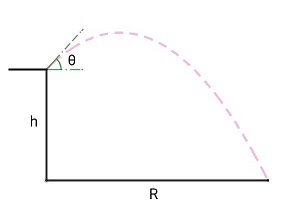# Maximizing Range 2A projectile is fired from a height $h$, as shown in the figure, with an initial velocity $v$ at an angle of inclination $\theta$ from the horizontal. Determine $\theta$ in degrees so that the range $R$ of the projectile, measured on the ground, is maximum.

Details and Assumptions

• $v^2=gh$.
• Neglect air resistance.
×

Problem Loading...

Note Loading...

Set Loading...